# Pulse-Width Modulation (PWM)

#### Pulse-Width Modulation — What is it?

A good definition of Pulse-Width Modulation (PWM) is already in the name itself. It means modulating/varying the width of the pulse only, not the frequency. In the digital control system, PWM is used to control the amount of power sent to a device. Therefore, the PWM is a very effective technique for controlling analog devices using the digital outputs of a microcontroller. It is widely used in many fields, such as measurement, communication, power control, and transformation.

##### Principle

A PWM signal consists of two main components that define its behavior: a duty cycle and a frequency.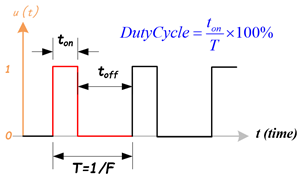• Duty Cycle
The duty cycle describes the amount of time the signal is in a high (on) state as a percentage of the total time it takes to complete one cycle. A lower duty cycle corresponds to lower power because the power is off most of the time. The duty cycle is expressed percent, 100% duty cycle would be fully on as same as setting the signal to Vcc (high); 0% duty cycle would be the same as grounding the signal.
• Frequency
The frequency determines how fast the PWM completes a cycle, ie. 100Hz would be 100 cycles per second. In other words, it shows how fast the PWM switches between high and low states.

In the digital system, PWM is the method to produce variable voltage using digital means. Typically, a digital system only has two output voltages, the high (5V, 3.3V … etc.) or the low (0V). But, how it is possible that a digital system can produce a voltage that is between the high and low voltages? Here, we use mathematics to analyze the PWM signal and the average output.

#### Mathematical Analysis of PWM signal

PWM uses a rectangular pulse wave whose pulse width is modulated resulting in the variation of the average value of the waveform. Consider a waveform such as shown in figure 2.Figure 2: A Pulse Wave

The pulse signal is f(t) with period T and a duty cycle D (0.0~1.0). The average voltage of the waveform is given by:Just focus on one cycle T, as f(t) is a pulse wave, its positive-pulse is for 0 < t < D×T, and zero-pulse is for D×T < t < T. The above expression then becomes:From this, it is obvious that the average value of the signal is directly dependent on the duty cycle D.

For example, if a digital signal produces a pulse high (5V) and a low (0V) equally, let us assume the signal in the high state for 1 microsecond and in a low state for 1 microsecond. The duty cycle is 50%, so the average voltage would be 2.5 volts. Now, change the high voltage in a high state for 7 microseconds and in a low state for 3 microseconds. The duty cycle now becomes to 70%, the average voltage would measure 70% of 5 volts or 5v x 0.7 = 3.5 volts.

#### Types of PWM

There are a couple of types of PWM, and they can be classified in different ways.

##### Classify PWM Signal by Methods: Symmetric and Asymmetric

There are two kinds of PWM signals: Symmetric and Asymmetric.

1. Symmetric PWM:
• The pulses of asymmetric PWM signals are always symmetric with respect to the center of each PWM period.
• Symmetric PWM is often used for three-phase AC induction and brushless DC motors, due to the lower harmonic distortion that is generated on phase currents in comparison to asymmetric PWM methods.
2. Asymmetric PWM:
• The pulses of an asymmetric PWM signal always have the same side aligned with one end of each PWM period.
• Asymmetric PWM can be used for stepper motors and other variable-reluctance motors.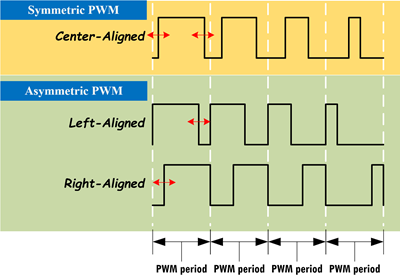Figure 3: Three Types of PWM Signal: Center-Aligned, Left-Aligned, and Right-Aligned PWMs

Classify PWM Signal by Signal-Alignment

The PWM signal can be classified by signal alignment in four different types:

1. Center-aligned PWM:
• Symmetric PWM
• Center-aligned PWMs are most often used in AC motor control to maintain phase alignment
2. Left-aligned PWM
• Asymmetric PWM
• Left-aligned PWMs are used for most general-purpose PWM uses
3. Right-aligned PWM
• Asymmetric PWM
• Right-aligned PWMs are typically only used in special cases that require alignment opposite of left-aligned PWMs
4. Dual-edge PWM
• Dual-edged PWMs are optimized for power conversion where phase alignment must be adjusted

#### Generating PWM with Microcontroller using Timer/Counter

The basic idea to generate a PWM signal is to use a counter (or timer), a CMP (compare) value, and a digital output pin. The counter continuously counts up or down and is compared with the CMP value. The digital output (PWM) will be changed when the counter matches the CMP value, or when the counter resets. It can be implemented by software or hardware. Most microcontrollers already have hardware modules that can generate PWM signals after initializing the registers.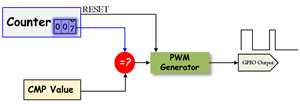#### PWM Timer

The PWM timer in the microcontroller runs in one of two modes: Count-Down mode or Count-Up/Down mode.

• In Count-Down mode, the timer counts from the Period (LOAD) value to zero, goes back to the Period (LOAD) value, and continues counting down.
• In Count-Up/Dow mode, the timer counts from zero up to the Period (LOAD) value, back down to zero, back up to the Period (LOAD) value, and so on.

Generally, the Count-Down mode is used for generating left- or right-aligned PWM signals, while the Count-Up/Down mode is used for generating center-aligned PWM signals.

Check the microcontroller's datasheet about the PWM timer. Most of the ARM microcontrollers use Count-Down mode.

## Center-Aligned PWM

A center-aligned PWM implements the PWM differently from all of the other modes. The PWM timer is configured in counting-up and -down mode. The counter starts at zero and counts up to the Period (LOAD) value, and when the Period (LOAD) value is reached, the counter starts counting back down to zero. In this mode, the Period (LOAD) value is actually half of the period of the final PWM output.

1. A single compare (CMP) value, which contains the duty cycle value, is constantly compared with the PWM timer (COUNTER) value. When the timer (COUNTER) value is less than the CMP value, the PWM output signal is de-asserted.
2. When the timer (COUNTER) value exceeds or is equal to the CMP value, the PWM output signal is asserted. When the timer (COUNTER) value reaches the Period (LOAD) value, the timer starts counting down to zero.
3. When the timer (COUNTER) value is less than or equal to the CMP value, the PWM output signal is de-asserted, and the process repeats.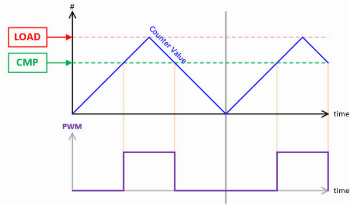#### PWM Module

The PWM module in the TI TIVA microcontroller generates PWM signals. Each PWM module contains four PWM Generators. Each generator can produce two PWM signals. Therefore, one PWM module totally can generate eight PWM signals.Figure 13.1: PWM Module Block Diagram

PWM Clock Configuration

The PWM module has two clock source options:

• The System Clock
• A pre divided System Clock

The clock source is selected by programming the USEPWMDIV bit in the Run-Mode Clock Configuration (RCC) register. The PWMDIV bit field specifies the divisor of the system clock that is used to create the PWM Clock.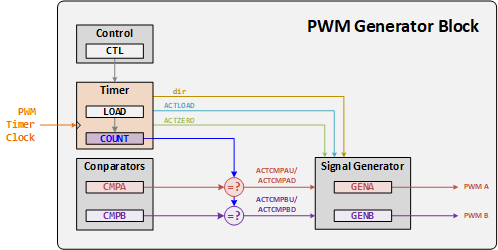Figure 13.2: PWM Generator Block Diagram

PWM Timer

The timer in each PWM generator runs in one of two modes: Count-Down mode or Count-Up/Down mode.

• In Count-Down mode, the timer counts from the LOAD value to zero, then goes back to the LOAD value, and continues counting down.
• In Count-Up/Down mode, the timer counts from zero up to the LOAD value, back down to zero, then back up to the LOAD value, and so on.

Generally, the Count-Down mode is used for generating left- or right-aligned PWM signals, while the Count-Up/Down mode is used for generating center-aligned PWM signals

PWM Comparators

Each PWM generator has two comparators that monitor the value of the counter, when either comparator matches the counter, they output a single-clock-cycle-width High pulse, labeled "ACTCMPA" and "ACTCMPB" in Figure 13.2. When in Count-Up/Down mode, these comparators match both when counting up and when counting down, and thus are qualified by the counter direction signal. These qualified pulses are used in the PWM generation process. If either comparator match value is greater than the counter load value, then that comparator never outputs a High pulse.

The timers output three signals that are used in the PWM generation process: the direction signal (this is always Low in Count-Down mode, but alternates between low and high in Count-Up/Down mode), a single-clock-cycle-width High pulse when the counter is zero, and a single-clock-cycle-width High pulse when the counter is equal to the LOAD value. Note that in Count-Down mode, the zero pulse is immediately followed by the load pulse. In the figures in this chapter, these signals are labeled "dir", "ACTZERO", and "ACTLOAD".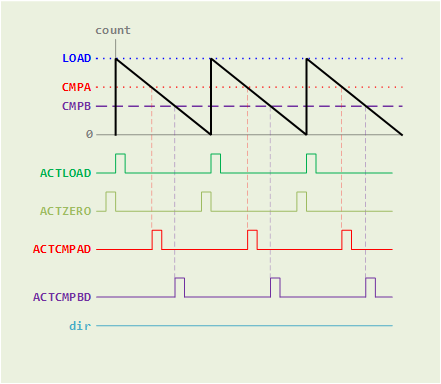Figure 13.3 (a): PWM Count-Down Mode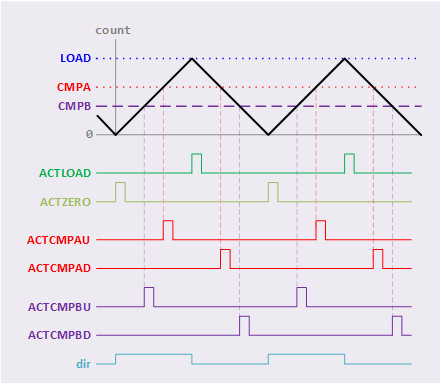Figure 13.3 (b): PWM Count-UP/Down Mode

PWM Signal Generator

Each PWM generator takes the ACTLOAD, ACTZERO, ACTCMPAD, ACTCMPAU, ACTCMPBD, and ACTCMPBU pulses (qualified by the dir signal) and generates two internal PWM signals, GENA and GENB.

• In Count-Down mode, there are four events that can affect these signals: ACTZERO, ACTLOAD, ACTCMPAD (match CMPA down), and ACTCMPBD (match CMPB down).
• In Count-Up/Down mode, there are six events that can affect these signals: ACTZERO, ACTLOAD, ACTCOMAD (match CMPA down), ACTCMPAU (match CMPA up), ACTCMPBD (match CMPB down), and ACTCMPBU (match CMPB up). The match A or match B events are ignored when they coincide with the ACTZERO or ACTLOAD events. If the match CMPA and match CMPB events coincide, the first signal, GENA, is generated based only on the match CMPA event, and the second signal, GENB, is generated based only on the match CMPB event.